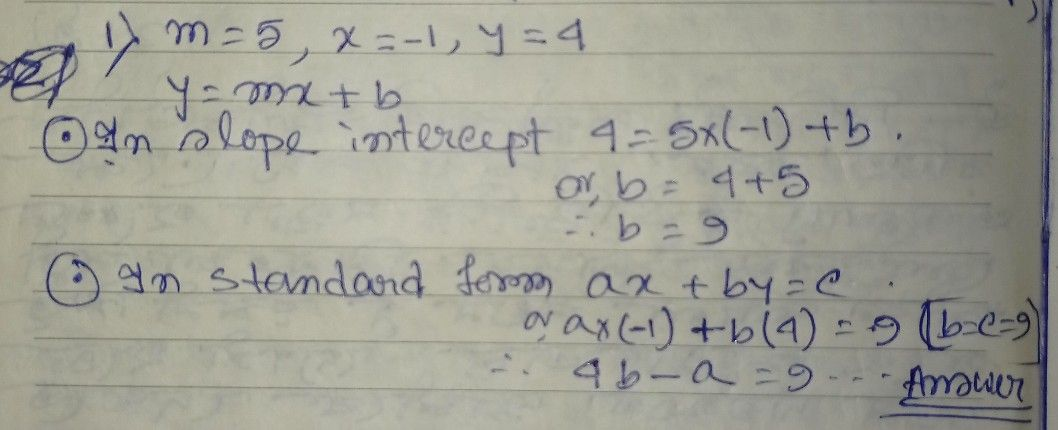Symbol
ProblemLearning Task $3$ Find the equation of the line, Do it in your notebook. (a) In slope intercept $fomy=mx+b$ (b) in standard form $a+by=c$ $11$ The slope is 5 passing through $\left(-1,4\right)$ 2. The line passes through point $\left(3,-4\right)$ and $nd$ $\left(-2,2\right)$ $3$ The slope is 4. and the $y-intercept$ is $\left(0,4\right)$ $4$ The x intercept $-3$ and the $y-intercept$ is 6 $5$ Passing through the points $\left(-1,-2\right)$ and $\left(5,$ $3\right)$
7th-9th grade
Other
Search count: 105
SolutionQanda teacher - AISHEE2603